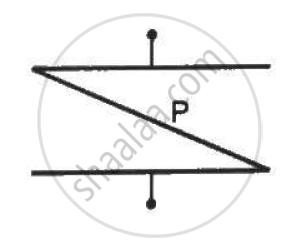Department of Pre-University Education, KarnatakaPUC Karnataka Science Class 12
Share

# A Thin Metal Plate P is Inserted Between the Plates of a Parallel-plate Capacitor of Capacitance C in Such a Way that Its Edges Touch the Two Plates . the Capacitance Now Becomes - Physics

ConceptCapacitors and Capacitance

#### Question

A thin metal plate P is inserted between the plates of a parallel-plate capacitor of capacitance C in such a way that its edges touch the two plates . The capacitance now becomes _________ .• C/2

• 2 C

• 0

• ∞

#### Solution

∞

The thin metal plate inserted between the plates of a parallel-plate capacitor of capacitance C connects the two plates of the capacitor; hence, the distance d between the plates of the capacitor reduces to zero. It can be observed that the charges on the plates begin to overlap each other via the metallic plate and hence begin to conduct continuously.

Mathematically ,

C = (∈_0A)/d

In this case , d = 0.

therefore C = ∞

Is there an error in this question or solution?

#### Video TutorialsVIEW ALL 

Solution A Thin Metal Plate P is Inserted Between the Plates of a Parallel-plate Capacitor of Capacitance C in Such a Way that Its Edges Touch the Two Plates . the Capacitance Now Becomes Concept: Capacitors and Capacitance.
S